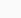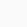# How to use power or superscript(a²,xⁿ) in LaTeX?

You need to use the bace^exponent command to print the superscript in a latex document.

Symbol/UnicodeSuperscript(Power)
Type of symbolMathematics
Package (requirement)No
ArgumentNo
Latex commanda^n
Examplea^2 → a²

## Use ^ symbol for superscript

Here a is the base and n is the exponent, use ^ key on the keyboard between base and exponent.

An excerpt of how to use it is shown below.

\documentclass{article}
\begin{document}
$\verb|a^n|\rightarrow a^n \quad \verb|a^3|\rightarrow a^3$
$\verb|sum^n|\rightarrow \sum^n \quad \verb|\prod^n|\rightarrow \prod^n$
$\verb|\Lambda^0|\rightarrow \Lambda^0 \quad \verb|\bigcap^n|\rightarrow \bigcap^n$
\end{document}

Output :## How to use more than one character as superscript

To use a character as a superscript, writing like this a^n is printed in the document an.

But when you want to use more than one character as a superscript then you have to enclose the characters in curly brackets {}. like this a^{mn}.

And if you don’t enclose the characters in curly brackets then the first character will be valid as a superscript and the remaining characters will be printed as normal text.

like this a^mnamn. Some more examples are given below for ease of understanding

\documentclass{article}
\begin{document}
$\verb|a^mn|\rightarrow a^mn \quad \verb|a^mng|\rightarrow a^mng$
$\verb|a^{mn}|\rightarrow a^{mn} \quad \verb|a^{mng}|\rightarrow a^{mng}$
$\verb|\sum^{\mu\beta}|\rightarrow \sum^{\mu \beta}$
\end{document}

Output :## Double or multiple superscripts in LaTeX

If you want to printthen you have to write like this a^{n^2}. Below are more examples of using multiple superscripts.

\documentclass{article}
\usepackage{yhmath}
\begin{document}
$a^{m^{n^g}} \quad a^{p^{q^r}}$
$\sum_{i=0}^n a_n = a^{a_1^{a_2^{a_3^{\adots^{a_n}}}}}$\end{document}

Output :Scroll to Top